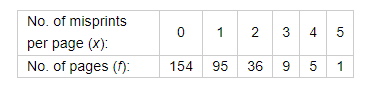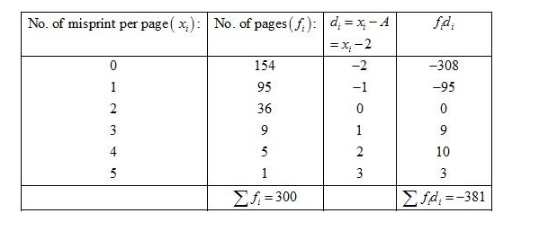# In the first proof reading of a book containing 300

Question:

In the first proof reading of a book containing 300 pages the following distribution of misprints was obtained:Find the average number of misprints per page

Solution:

Let the assume mean be $A=2$.We know that mean, $\bar{X}=A+\frac{1}{N} \sum_{i=1}^{n} f_{i} d_{i}$

Now, we have $N=\sum f_{i}=300, \sum f_{i} d_{i}=-381$ and $A=2$.

Putting the values in above formula, we have

$\bar{X}=A+\frac{1}{N} \sum_{i=1}^{n} f_{i} d_{i}$

$=2+\frac{1}{300} \times(-381)$

$=2-\frac{381}{300}$

$=2-1.27$

$=0.73$

Hence, the mean number of students absent per day is 0.73.